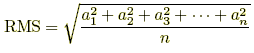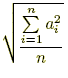index: click on a letter A B C D E F G H I J K L M N O P Q R S T U V W X Y Z A to Z index index: subject areas numbers & symbols sets, logic, proofs geometry algebra trigonometry advanced algebra & pre-calculus calculus advanced topics probability & statistics real world applications multimedia entrieswww.mathwords.com about mathwords website feedback

Root Mean Square
RMS

A kind of average sometimes used in statistics and engineering, often abbreviated as RMS. To find the root mean square of a set of numbers, square all the numbers in the set and then find the arithmetic mean of the squares. Take the square root of the result. This is the root mean square.

 Formula:orExample: For the numbers 4 and 9,